# Color With C#

---
date: Jun 12, 2016
tags:
- C#
language: English
---

Recently, i am doing a research on a project to convert RGB to HSB, which leads me to an awesome article on CodeProject from 9 years ago. Hence, I sort the article a little bit to help myself understand more of color agorithm.

### Index

What is “Color”?

Color models

Conversion between models

Using the code

### What is “Color”?

"Color is the visual perceptual property corresponding in humans to the categories called red, yellow, white, etc. Color derives from the spectrum of light (distribution of light energy versus wavelength) interacting in the eye with the spectral sensitivities of the light receptors. Color categories and physical specifications of color are also associated with objects, materials, light sources, etc., based on their physical properties such as light absorption, reflection, or emission spectra.

Color definition, Wikipedia.

Colorimetry is the science that describes colors in numbers, or provides a physical color match using a variety of measurement instruments. Colorimetry is used in chemistry, and in industries such as color printing, textile manufacturing, paint manufacturing and in the food industry.

Colorimetry definition, Wikipedia.

Then, how can we display colors as numbers? the answer: color models.

### Color Models

#### RGB (Red Green Blue)

The RGB (Red, Green, Blue) color model is the most known, and the most used every day. It defines a color space in terms of three components:

• Red, which ranges from 0-255
• Green, which ranges from 0-255
• Blue, which ranges from 0-255

The RGB color model is an additive one. In other words, Red, Green and Blue values (known as the three primary colors) are combined to reproduce other colors.

For example, the color “Red” can be represented as [R=255, G=0, B=0], “Violet” as [R=238, G=130, B=238], etc.

Its common graphic representation is the following image: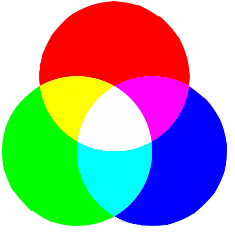In .NET, the Color structure use this model to provide color support through R, G and B properties.

but this is not its only usage. For this reason, we can define a dedicated RGB structure for further coding, as shown below:

#### HSB color space

The HSB (Hue, Saturation, Brightness) color model defines a color space in terms of three constituent components:

• Hue : the color type (such as red, blue, or yellow).

• Ranges from 0 to 360° in most applications. (each value corresponds to one color : 0 is red, 45 is a shade of orange and 55 is a shade of yellow).
• Saturation : the intensity of the color.

• Ranges from 0 to 100% (0 means no color, that is a shade of grey between black and white; 100 means intense color).
• Also sometimes called the “purity” by analogy to the colorimetric quantities excitation purity.
• Brightness (or Value) : the brightness of the color.

• Ranges from 0 to 100% (0 is always black; depending on the saturation, 100 may be white or a more or less saturated color).

Its common graphic representation is the following image: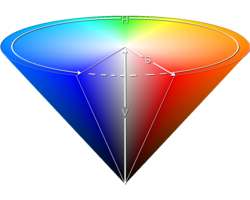The HSB model is also known as HSV (Hue, Saturation, Value) model. The HSV model was created in 1978 by Alvy Ray Smith. It is a nonlinear transformation of the RGB color space. In other words, color is not defined as a simple combination (addition/substraction) of primary colors but as a mathematical transformation.

Note: HSV and HSB are the same, but HSL is different.

All this said, a HSB structure can be :

#### HSL color space

The HSL color space, also called HLS or HSI, stands for:

• Hue : the color type (such as red, blue, or yellow).
• Ranges from 0 to 360° in most applications (each value corresponds to one color : 0 is red, 45 is a shade of orange and 55 is a shade of yellow).
• Saturation : variation of the color depending on the lightness.
• Ranges from 0 to 100% (from the center of the black&white axis).
• Lightness (also Luminance or Luminosity or Intensity).
• Ranges from 0 to 100% (from black to white). Its common graphic representation is the following image: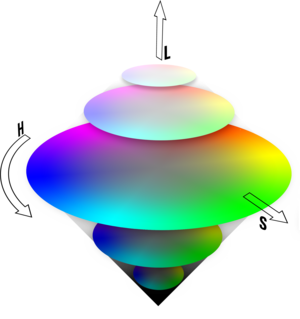HSL is similar to HSB. The main difference is that HSL is symmetrical to lightness and darkness. This means that:

• In HSL, the Saturation component always goes from fully saturated color to the equivalent gray (in HSB, with B at maximum, it goes from saturated color to white).
• In HSL, the Lightness always spans the entire range from black through the chosen hue to white (in HSB, the B component only goes half that way, from black to the chosen hue).

For my part, HSL offers a more accurate (even if it’s not absolute) color approximation than HSB. All this said, a HSL structure can be:

#### CMYK color space

The CMYK color space, also known as CMJN, stands for:

• Cyan.
• Ranges from 0 to 100% in most applications.
• Magenta.
• Ranges from 0 to 100% in most applications.
• Yellow.
• Ranges from 0 to 100% in most applications.
• blacK.
• Ranges from 0 to 100% in most applications.

It is a subtractive color model used in color printing.CMYK works on an optical illusion that is based on light absorption.

The principle is to superimpose three images; one for cyan, one for magenta and one for yellow; which will reproduce colors. Its common graphic representation is the following image: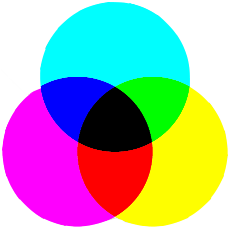Like the RGB color model, CMYK is a combination of primary colors (cyan, magenta, yellow and black). It is, probably, the only thing they have in common.

CMYK suffers from a lack of color shades that causes holes in the color spectrum it can reproduce. That’s why there are often differencies when someone convert a color between CMYK to RGB.

Why using this model? Why black is used? you can tell me… Well it’s only for practical purpose. Wikipedia said:

• To improve print quality and reduce moiré patterns,
• Text is typically printed in black and includes fine detail (such as serifs); so to reproduce text - using three inks would require an extremely precise alignment for each three components image.
• A combination of cyan, magenta, and yellow pigments don’t produce (or rarely) pure black.
Mixing all three color inks together to make black can make the paper rather wet when not using dry toner, which is an issue in high speed printing where the paper must dry extremely rapidly to avoid marking the next sheet, and poor quality paper such as newsprint may break if it becomes too wet.
• Using a unit amount of black ink rather than three unit amounts of the process color inks can lead to significant cost savings (black ink is often cheaper).

Let’s come back to our reality. A CMYK structure can be:

#### YUV color space

The YUV model defines a color space in terms of one luma and two chrominance components. The YUV color model is used in the PAL, NTSC, and SECAM composite color video standards.

YUV models human perception of color more closely than the standard RGB model used in computer graphics hardware.

The YUV color space stands for:

• Y, the luma component, or the brightness.
• Ranges from 0 to 100% in most applications.
• U and V are the chrominance components (blue-luminance and red-luminance differences components).
• Expressed as factors depending on the YUV version you want to use.

A graphic representation is the following image: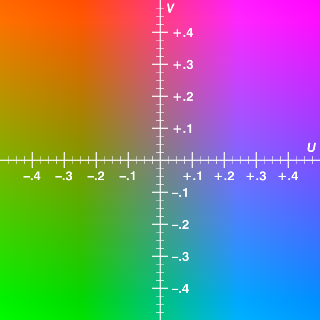A YUV structure can be:

#### CIE XYZ color space

In opposition to the previous models, the CIE XYZ model defines an absolute color space. It is also known as the CIE 1931 XYZ color space and stands for:

• X, which can be compared to red
• Ranges from 0 to 0.9505
• Y, which can be compared to green
• Ranges from 0 to 1.0
• Z, which can be compared to blue
• Ranges from 0 to 1.089

Before trying to explain why I include this color space in this article, you have to know that it’s one of the first standards created by the International Commission on Illumination (CIE) in 1931. It is based on direct measurements of the human eye, and serves as the basis from which many other color spaces are defined.

A graphic representation is the following image: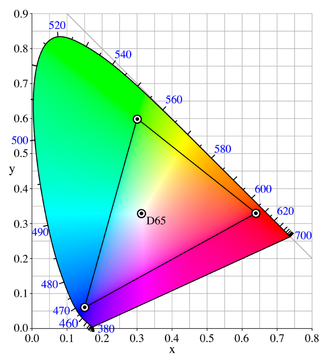A CIE XYZ structure can be:

Well! why do I have to include this model?

I have made a quick research to include Cie L*ab color model in this article, and I find that a conversion to an absolute color space is required before converting to L*ab. The model used in the conversion principle is Cie XYZ. So, I’ve included it and now everyone can understand “what are those XYZ values” used further in the article.

#### CIE Lab color space

A Lab color space is a color-opponent space with dimension L for luminance and a and b for the color-opponent dimensions, based on nonlinearly-compressed CIE XYZ color space coordinates."

As said in the previous definition, CIE Lab color space, also know as CIE 1976 color space, stands for:

• L*, the luminance
• a*, the red/green color-opponent dimension
• b* , the yellow/blue color-opponent dimension

The Lab color model has been created to serve as a device independent model to be used as a reference. It is based directly on the CIE 1931 XYZ color space as an attempt to linearize the perceptibility of color differences.

The non-linear relations for L*, a*, and b* are intended to mimic the logarithmic response of the eye, coloring information is referred to the color of the white point of the system.

A CIE Lab structure can be:

There are still many other formats like RYB and CcMmYK. I still don’t intend to create a “color framework”(so do I), but if you have other ideas…

### Conversion between models

#### A - RGB Conversions

Converting RGB color to any other model is the basis in conversion algorithms. It implies a normalisation of red, green and blue : value ranges now from [0…255] to [0…1].

##### a - RGB to HSB

The conversion principle is the one below:

H ? [0, 360]

S, V, R, G, B ? [0, 1]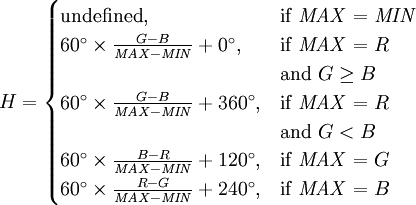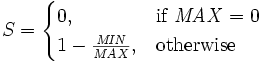V = MAX

Well! Interesting! But what’s the C# equivalent? Here it is.

##### b - RGB to HSL

The conversion principle is the one below:

H ? [0, 360]

S, L, R, G, B ? [0, 1]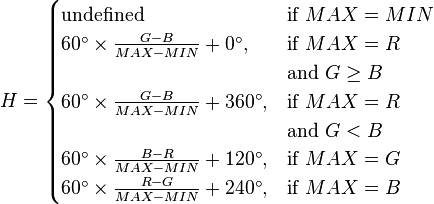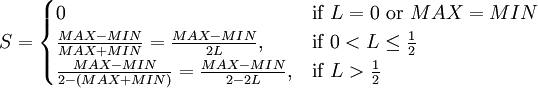L = 1/2*(MAX + MIN)

The C# equivalent is:

Note: You have probably noticed String.Format in the final line. It’s the .NET solution for keeping the same rounding behavior. If you don’t understand what I mean, try the sample code below:

You didn’t notice a problem? Ok, rouding 4.45 should have returned 4.5 and not 4.4. The solution is using `String.Format()` which always applies “round-to-even” method.

##### c - RGB to CMYK

The conversion principle is the one below :

R, G, B ? [0, 1]

t**C’M’Y’ = {1 - R, 1 - G, 1 - B}

K = min{C’, M’, Y’}

t**CMYK = {0, 0, 0, 1}

if K = 1tCMYK = { (C’ - K)/(1 - K), (M’ - K)/(1 - K), (Y’ - K)/(1 - K), K } otherwise

The C# equivalent is:

##### d - RGB to YUV (YUV444)

The conversion principle is the one below :

R, G, B, Y ? [0, 1]

U ? [-0.436, 0.436]

V ? [-0.615, 0.615]

tYUV = { (0.299 R + 0.587 G + 0.114 B), (- 0.14713 R + 0.28886 G + 0.436 B), (0.615 R + 0.51499 G + 0.10001 B) }

The C# equivalent is :

##### e - RGB to web color

Haaa! Something I can explain.

As you probably already know, web colors can be defined in two ways: for example, “red” can be defined as `rgb(255,0,0)` or `#FF0000`.

The explanation of the second form is simple :

• `#`” character tells that the format is the hexadecimal one.
• The last 6 characters define 3 pairs: one for “Red”, one for “Green” and one for “Blue”.
• Each pair is a hexadecimal value (base 16) of a value which ranges from 0 to 255.

So, you can divide each color component by 16 and replace numbers superior to 9 by theirs hexadecimal value (eg. 10 = A, 11 = B, etc.)… but the best way is to use `String.Format()` habilities.

##### f - RGB to XYZ

The conversion principle is the one below:

R, G, B ? [0, 1]

a = 0.055 and ? ˜ 2.2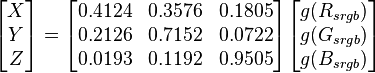where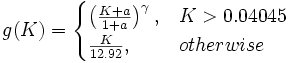The C# equivalent is :

##### g - RGB to Lab

As I said before, converting to the CIE Lab color model is a little bit tricky: we need to convert to CIE XYZ before trying to have Lab values.

The conversion between XYZ and Lab is given below.

#### B - HSB conversions

##### a - HSB to RGB

The conversion principle is the one below :

H ? [0, 360]

S, V, R, G, B ? [0, 1]

Hi = [H / 60] mod 6

f = (H / 60) - Hi

p = V (1 - S)

q = V (1 - f S)

t = V (1 - (1 - f ) S)

if Hi = 0 ? R = V, G = t, B = p
if Hi = 1 ? R = q, G = V, B = p
if Hi = 2 ? R = p, G = V, B = t
if Hi = 3 ? R = p, G = q, B = V
if Hi = 4 ? R = t, G = p, B = V
if Hi = 5 ? R = V, G = p, B = q

The C# equivalent? Here it is.

##### b - HSB to HSL

Nothing new: conversion principle is to convert to RGB and then to HSB.

##### c - HSB to CMYK

Nothing new: conversion principle is to convert to RGB and then to CMYK.

##### d - HSB to YUV

Nothing new: conversion principle is to convert to RGB and then to YUV.

#### C - HSL conversions

##### a - HSL to RGB

The conversion principle is the one below :

H ? [0, 360]
S, L, R, G, B ? [0, 1]

if L < 0.5 ? Q = L × (1 + S)
if L = 0.5 ? Q = L + S – (L × S)

P = 2 × LQ

Hk = H / 360

Tr = Hk + 1/3

Tg = Hk

Tb = Hk – 1/3

For each c = R,G,B :

if Tc < 0 ? Tc = Tc + 1.0
if Tc > 1 ? Tc = Tc – 1.0

if Tc < 1/6 ? Tc = P + ((QP) × 6.0 × Tc)
if 1/6 = Tc > 1/2 ? Tc = Q
if 1/2 = Tc > 2/3 ? Tc = P + ((QP) × (2/3 – Tc) × 6.0)
else Tc = P

The C# equivalent? Here it is.

##### b - HSL to HSB

Nothing new: conversion principle is to convert to RGB and then to HSB.

##### c - HSL to CMYK

Nothing new: conversion principle is to convert to RGB and then to CMYK.

##### d - HSL to YUV

Nothing new: conversion principle is to convert to RGB and then to YUV.

#### D - CMYK conversions

##### a - CMYK to RGB

The conversion principle is the one below :

tRGB = { (1 - C) × (1 - K) , (1 - M) × (1 - K), (1 - Y) × (1 - K)}

The C# equivalent? Here it is.

##### b - CMYK to HSL

Nothing new: conversion principle is to convert to RGB and then to HSL.

##### c - CMYK to HSB

Nothing new: conversion principle is to convert to RGB and then to HSB.

##### d - CMYK to YUV

Nothing new: conversion principle is to convert to RGB and then to YUV.

#### E - YUV conversions

##### a - YUV to RGB

The conversion principle is the one below :

R, G, B, Y ? [0, 1]

U ? [-0.436, 0.436]

V ? [-0.615, 0.615]

tRGB = { (Y + 1.13983 V), (Y - 0.39466 U - 0.58060 V), (Y + 2.03211 U) }

The C# equivalent? Here it is.

##### b - YUV to HSL

Nothing new: conversion principle is to convert to RGB and then to HSL.

##### c - YUV to HSB

Nothing new: conversion principle is to convert to RGB and then to HSB.

##### d - YUV to CMYK

Nothing new: conversion principle is to convert to RGB and then to CMYK.

#### F - XYZ conversions

##### a - XYZ to RGB

The conversion principle is the one below:

a = 0.055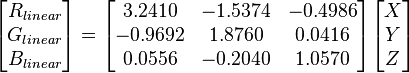then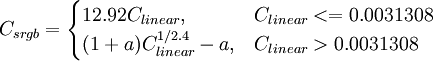The C# equivalent is :

##### b - XYZ to Lab

The conversion principle is the one below: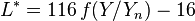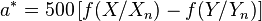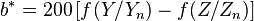where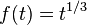for t > 0.008856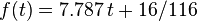otherwise

Xn, Yn and Zn are the CIE XYZ tristimulus values of the reference white point.

The C# equivalent is:

#### G - La*b* conversions

##### a - La*b* to XYZ

The conversion principle is the one below:

d = 6/29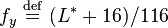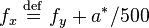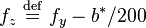if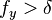then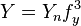else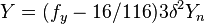if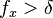then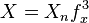else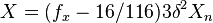if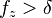then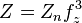else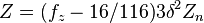The C# equivalent is:

##### b - La*b* to RGB

Nothing really new, the principle is to convert to XYZ and then to RGB :

### Using the code

Well, after showing you the conversion algorithms, maybe there is nothing more I can tell you.

In fact, there are many other useful methods in `ColorSpaceHelper`. You will find:

• Average color implementation (`ColorSpaceHelper.GetColorDistance()`).
• Wheel color generation (`ColorSpaceHelper.GetWheelColors()`) with 32bit support (alpha).
• Light spectrum color generation for (`ColorSpaceHelper.GetSpectrumColors()`) with 32bit support (alpha).
• Conversion to and from web colors (`ColorSpaceHelper.HexToColor()`).
• Conversion to and from `System.Drawing.Color` and the other structures.

Also, I am planning to write a C# class for colors for easier use in UWP and WPF apps. Hope you like it! link(not finished)